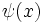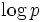# Second Chebyshev function

View other such summations

## Definition

Letbe a positive real number. The second Chebyshev function of, denoted, is defined as the following sum:.

Here,is the von Mangoldt function.

This summation is taken over all the natural numbers less than or equal to; however, a positive contribution comes only from prime powers, and the contribution of a prime poweris.

## Relation with other functions

### Exponential

The exponential of the second Chebyshev function gives the lcm of all numbers so far. In other words:wheredenotes the greatest integer function of, i.e., the largest integer less than or equal to.